# How To Find A Electron Configuration For Nitrogen

Nitrogen is one of the chemical element that has a symbol N. The atomic number of nitrogen is 7. A Scottish physician Danial Rutherford discovered and isolated nitrogen in the year 1772. However, Henry Cavendish and Carl Wilhelm Scheele had independently during the same time but Rutherford is generally accorded the credit and appreciated as his work got published first. In this article today we are going to tell you all the things about nitrogen.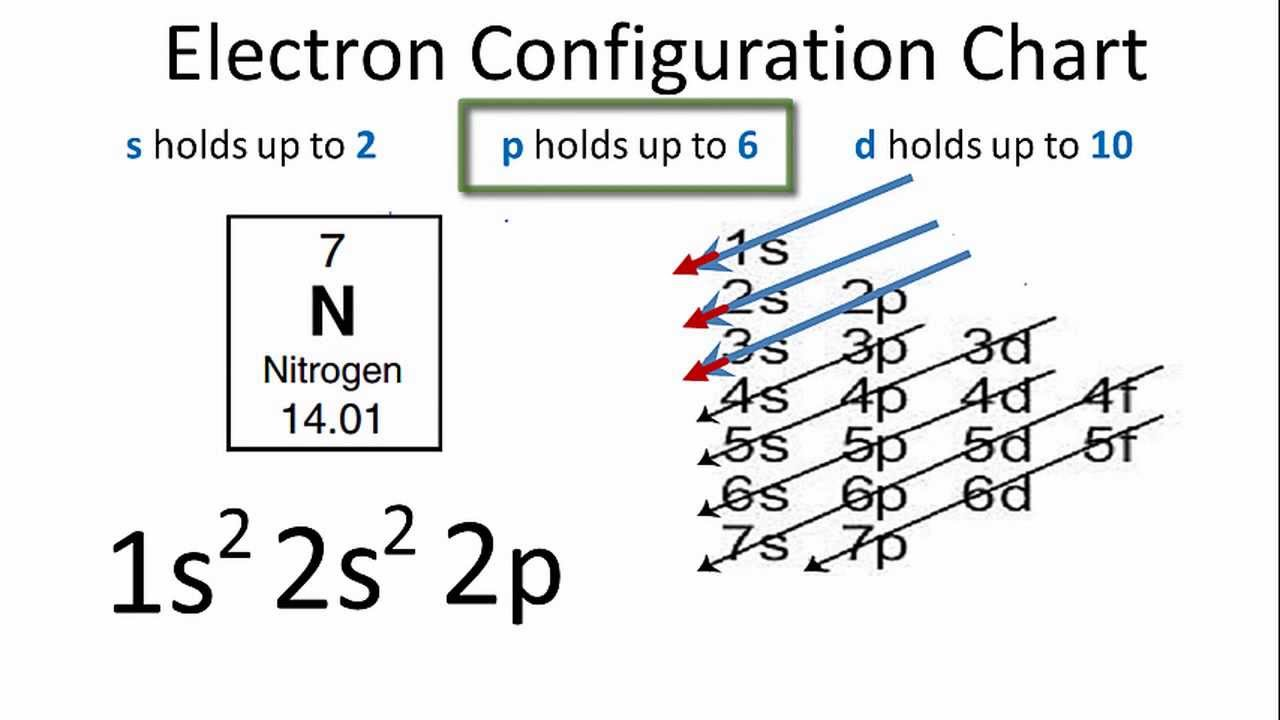### Electron Configuration For Nitrogen Ion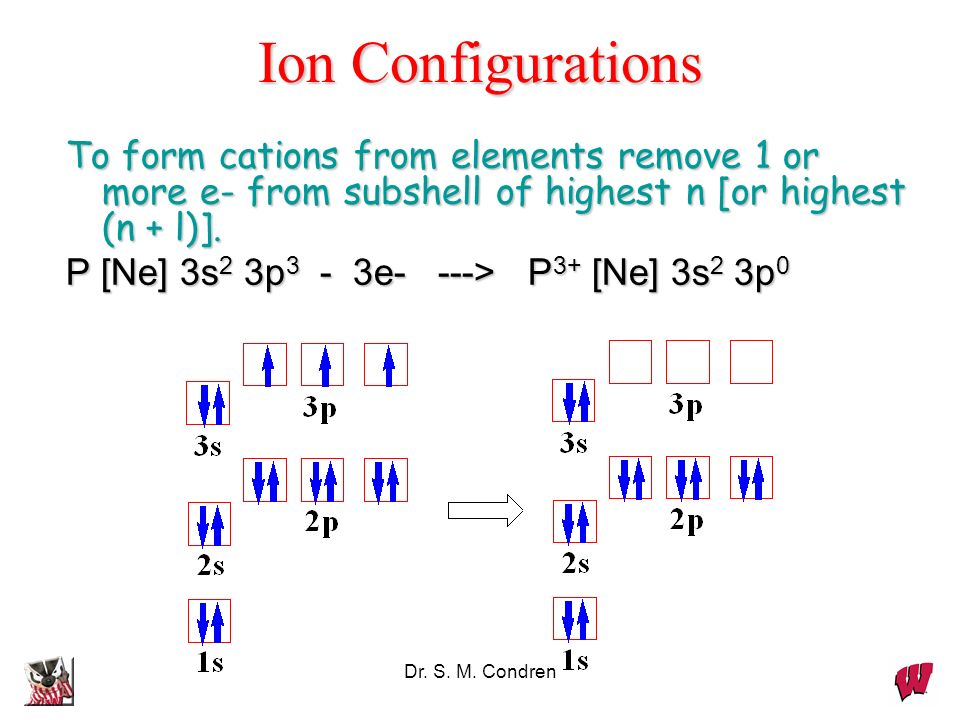Nitrogen is the seventh element of the periodic table with a total of 7 electrons. When we write the electron configuration of nitrogen the first two electrons go in the 1s orbital. As 1s can only hold 2 electrons and the other next two electrons for Nitrogen (N) go in the 2s orbital. The three electrons that are remained will go in the 2p orbital. Therefore the Nitrogen electron configuration is 1s22s22p3.

### Nitrogen Number of Valence Electrons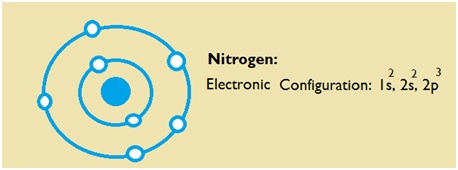Nitrogen has five valence electron in its outer shell.

### Ground State Electron Configuration for Nitrogen

1s22s22p3 is the ground state electron configuration for Nitrogen. You can easily understand the image given below.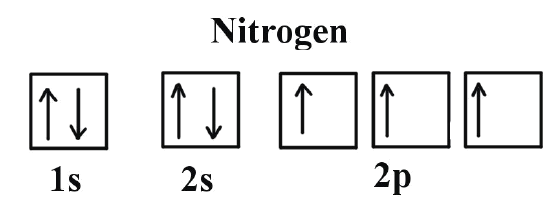### What is the Electron Configuration of Nitrogen

The electron configuration of nitrogen is 1s2 2s2 2p3. You can see the image below.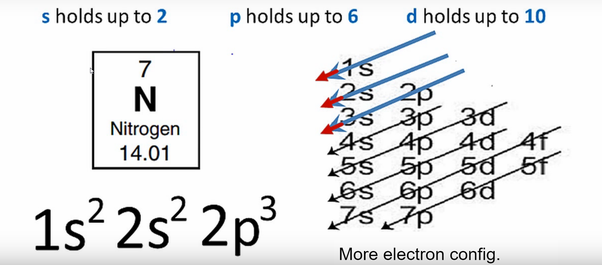### What is the Orbital Diagram for Nitrogen?

For drawing the orbital diagram configuration of the nitrogen we have to write the following:

The first 2 electrons pair up in the 1s orbital and the next two electrons pair up in the 2s orbital.